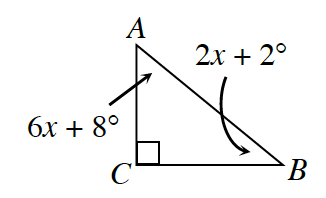### Home > GC > Chapter 4 > Lesson 4.1.3 > Problem4-29

4-29.Use what you know about the sum of the angles of a triangle to find $m∠ABC$ and $m∠BAC$. Are these angles acute or obtuse? Find the sum of these two angles. How can you describe the relationship of these two angles?

What is the relationship among the angles in a triangle? Use this relationship to write an equation and solve for $x$.